# Simple Harmonic Motion

### 7. Total energy in SHM

• When a system at rest is displaced from its equilibrium position by doing work on it, it gains potential energy and when it is released, it begins to move with a velovity and acquires kinetic energy.
• If m is the mass of system executing SHM then kinetic energy of system at any instant of time is
K=(1/2)mv2                     (13)
putting equation 8 in 13 we get,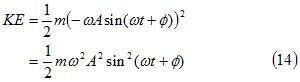• From equation (14) we see that Kinetic Energy of system varies periodically i.e., it is maximum (= (1/2)mω2A2) at the maximum value of velocity ( ±ωA) and at this time displacement is zero.
• When displacement is maximum (±A), velocity of SHM is zero and hence kinetic energy is also zero and at these extreme points where kinetic energy K=0, all the energy is potential.
• At intermediate positions of lying between 0 and ±A, the energy is partly kinetic and partly potential.
• To calculate potential energy at instant of time consider that x is the displacement of the system from its equilibrium at any time t.
• We know that potential energy of a system is given by the amount of work required to move system from position 0 to x under the action of applied force.
• Here force applied on the system must be just enough to oppose the restoring force -kx i.e., it should be equal to kx.
• Now work required to give infinitesimal displacement is dx=kx dx.
Thus, total work required to displace the system from 0 to x is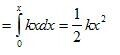thus,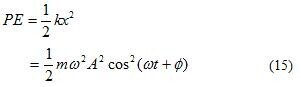where, from equation 5 ω=√(k/m) and displacement x=A cos(ωt+φ).
-From equation 14 and 15 we can calculate total energy of SHM which is given by,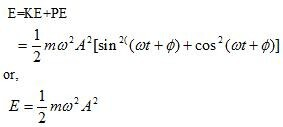• Thus total energy of the oscillator remains constant as displacement is regained after every half cycle.
• If no energy is dissipated then all the potential energy becomes kinetic and vice versa.
• Figure below shows the variation of kinetic energy and potential energy of harmonic oscillator with time where phase φ is set to zero for simplicity.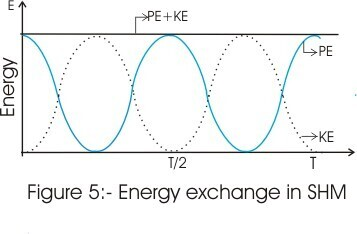## 8. Some simple systems executing SHM

### (A) Motion of a body suspended from a spring

• Figure (6a) below shows a spring of negligible mass, spring constant k and length l suspended from a rigid support.
• When a body of mass m is attached to this spring as shown in figure 6(b), the spring elongates and it would then rest in equilibrium position such that upward force Fup exerted by spring is equal to the weight mg of the boby.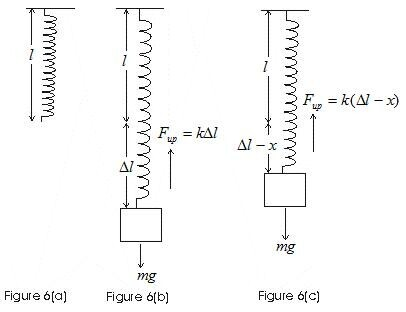• If the spring is extended by an amount Δl ater attachment of block of mass m then in its equilibrium position upward force equals
Fup=kΔl
also in this equilibrium position
Fup=mg
or,      kΔl=mg
• Again the body is displaced in upwards direction such that it is at a distance x above equilibrium position as shown in figure 6(c).
• Now extansion of spring would be (Δl-x), thus upward force now exerted on the body is
Fup=k(Δl-x)
• Weight of the body now tends to pull the spring downwards with a force equal to its weight. Thus resultant force on the body is
F=k(Δl-x)-mg
=mg-kx-mg

or,
F=-kx                     (17)
• From equation 17 we see that resultant force on the body is proportional to the displacement of the body from its equilibrium position.
• If such a body is set into vertical oscillations it oscillates with an angular frequency
•      ω=√(k/m)          (18)